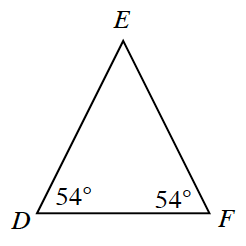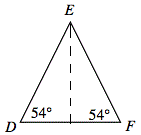### Home > INT2 > Chapter 4 > Lesson 4.2.1 > Problem4-65

4-65.

Lizzie notices that two angles in $ΔDEF$, shown at right, have the same measure.1. Based on this information, what statement can you make about the relationship between $\overline{ED}$ and $\overline{EF}$?

What's true about sides opposite equal angles in a triangle?

2. Determine the length of $\overline{DF}$ if $DE=9$ mm.

Divide $ΔDEF$ into two congruent right triangles.Use trigonometry to determine $DF$.